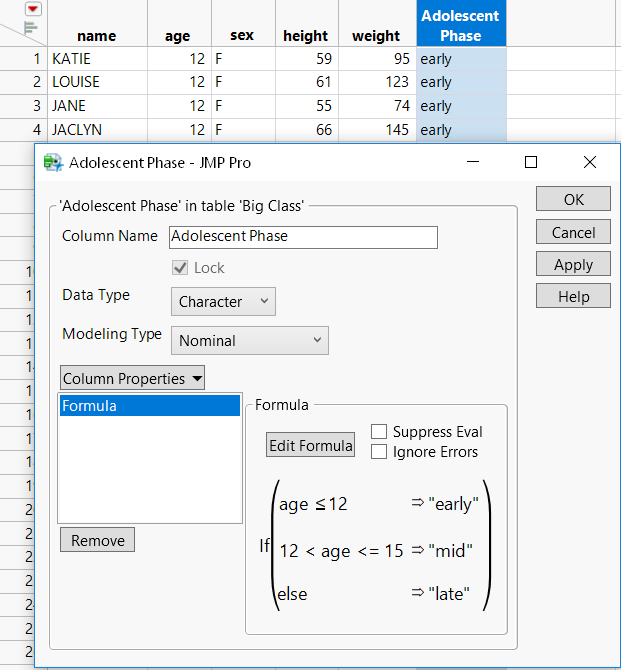Scripting Guide > Common Tasks > Create a Formula Column
Publication date: 11/10/2021

# Create a Formula Column

How do you create a formula column that combines conditional expressions with value comparisons? Create a Formula Column.jsl shows how to create a new formula column that evaluates ages in Big Class.jmp and returns the result in the new column (Figure 17.3).

/* Scenario:

` `

How do you create a formula column that combines conditional expressions

with value comparisons? This script shows how to create a new formula

column that evaluates ages in Big Class.jmp and returns the conditional

result in the new column. */

` `
`dt = Open( "\$SAMPLE_DATA/Big Class.jmp" );`
` `

/* Create a new character column for the formula.

Insert "early" in the new column if the age is less than or

equal to 12. Insert "mid" if the age is less than or equal to

15 but greater than 12. For ages greater than 15, insert "late".

*/

` `
`dt << New Column( "Adolescent Phase",`
`	Character,`
`	Formula(`
`		If( :age <= 12, "early",`
`			12 < :age <= 15, "mid",`
`			"late"`
`			)`
`		)`
`);`

Figure 17.3 Conditional Expression in Formula